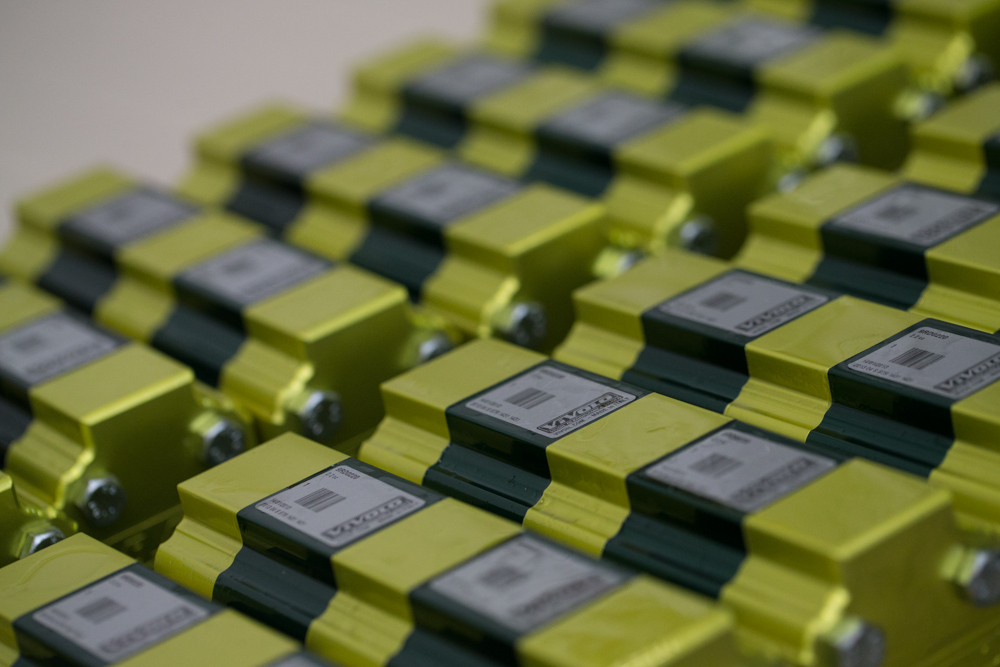# Use a Vivoil Flow Divider as Pressure MultipliersFlow dividers are very high precision hydraulic components, which are normally used to distribute the oil flow in several uses. However, this is not the only possible application. In some cases, in fact, it may be useful to choose to use a flow divider as a pressure multipliers.

Let’s see together when to opt for this particular solution and what benefits can be obtained.

## When to use a divider as a pressure multipliers?

This application of the flow divider is useful if you want to optimize the energy consumption of a system and reduce its waste, simply by discharging part of the oil. So, for example, you can use the residual energy at low pressure already available in your system, without having to create a separate line, obtaining a lower flow rate at a higher pressure.

It is a useful function in many cases and in many sectors, because Vivoil products are essential components of machines and systems used daily in different working environments:

## Which flow dividers can be used as pressure multipliers?

It is important to clarify that not all flow dividers can be used as pressure multipliers.

Those most used for this function are the models without valves.

It is possible to use flow dividers with valves, which in that case allow at the same time to limit the amplification according to the pressure at which the valve is regulated.

In general, it is important that the pressure obtained through this multiplication is kept below the maximum allowed for the specific type of divider (which you can check in our catalogs).

This is therefore an option that can be used with the majority of  Vivoil hydraulic flow dividers, except for one, i.e. the MIA-FD, because it contains valves that compensate for pressure differences and do not allow multiplication.

The MIA-FD (Manifold Istantaneous Auto-compensating Flow Divider) is the new generation of Vivoil dividers that distributes and feeds the different branches of the circuits with constant and independent flow rates. So, unlike the other components of this type, which are also useful as pressure multipliers, the MIA-FD is indicated when the uses are strongly unbalanced, for example if you have to handle differences in load, as in the lifting of trucks – heavier at the front and lighter at the back – or livestock platforms, because during the movements the animals move and therefore continuously unbalance the weight.

Do you want to use a Vivoil flow divider as a pressure multiplier?